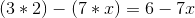Calculus 3 : Matrices

Example Questions

Example Question #131 : Matrices

Find the determinant of the 3x3 matrix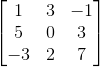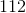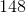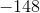Explanation:

To find the determinant of any 3x3 matrix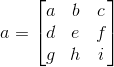, we use the formula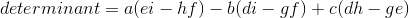. Using the vector from the problem statement, we get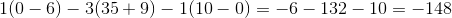Example Question #132 : Matrices

Perform the matrix operation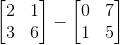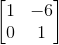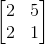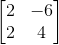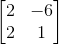Explanation:

To find the difference between two 2x2 matrices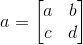and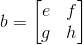you use the formula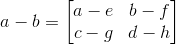. Using the two matrices from the problem statement, we then get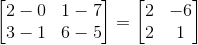Example Question #133 : Matrices

Perform the matrix operation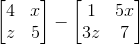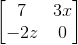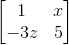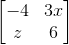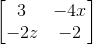Explanation:

To find the difference between two 2x2 matricesandyou use the formula. Using the two matrices from the problem statement, we then get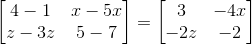Example Question #134 : Matrices

Find the determinant of the 2x2 matrix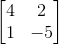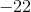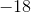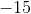Explanation:

To find the determinant of a 2x2 matrix,we use the formula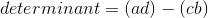Using hte matrix from the problem statement, we get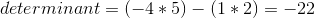Example Question #135 : Matrices

Find the determinant of the 3x3 matrix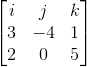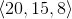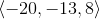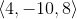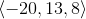Explanation:

To find the determinant of the 3x3 matrix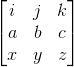, we use the formula: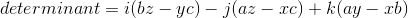. Using the matrix from the problem statement, we get: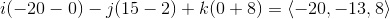Example Question #136 : Matrices

Find the determinant of the 3x3 matrix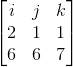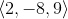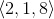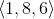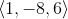Explanation:

To find the determinant of the 3x3 matrix, we use the formula:. Using the matrix from the problem statement, we get: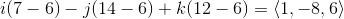Example Question #137 : Matrices

Perform the matrix operation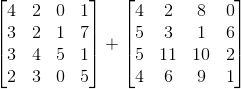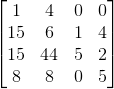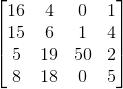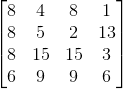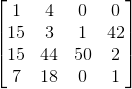Explanation:

To do matrix summation, you apply the following formula, where a and b are 4x4 matrices: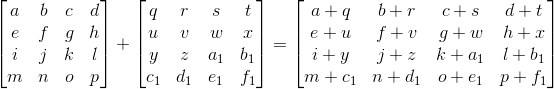Using this formula, we getExample Question #138 : Matrices

Find the determinant of the 3x3 matrix given: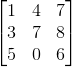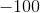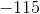Explanation:

To find the the determinant of a 3x3 matrix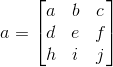, you apply the following formula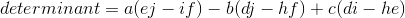Applying to the matrix from the problem statement, we get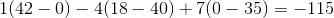Example Question #139 : Matrices

Find the determinant of the 3x3 matrix given: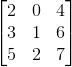Explanation:

To find the the determinant of a 3x3 matrix, you apply the following formulaApplying to the matrix from the problem statement, we get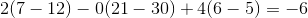Example Question #140 : Matrices

Find the determinant of the 2x2 matrix given: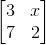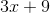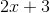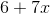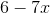Explanation:

To find the determinant of a 2x2 matrix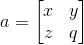, we use the formula: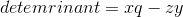Using the vector from the problem statement, we get: